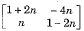# if A and B be real symmetric matrices of sizen nxn, then

1.  AT=1

2.  A=A-1

3.  AB=BA

4.  (AB)T=BA

4

(AB)T=BA

Explanation :
No Explanation available for this question

# rank of the matrix is

1.  0

2.  1

3.  2

4.  3

4

1

Explanation :
No Explanation available for this question

# consider the following statements S1:sum of the two singular n n matrices may be non-singular S2: sum of the two non-singular n n matrices may be singular. Which of the following statements is correct

1.  S1 and S2 are both true

2.  S1 is true, S2 is false

3.  S1 is false,S2 is true

4.  S1and S2 are both false

4

S1 and S2 are both true

Explanation :
No Explanation available for this question

# rank of the matrix is

1.  3

2.  1

3.  2

4.  4

4

4

Explanation :
No Explanation available for this question

# Let A=(aij) be an n-rowed square matrix and I12 be the matrix obtained by interchanging the first and second rows of the n-rowed identity matrix. Then AI12 is such that its first

1.  Row is the same as its second row

2.  Row is the same as the second row of A

3.  Column is the same as the second column of A

4.  Row is all zero

4

Column is the same as the second column of A

Explanation :
No Explanation available for this question

# let Ax=b be a system of linear equations, where A is an mxn matrix and b is an mx1 column vector and x is an nx1 column vector of unknowns.Which of the following is false

1.  The system has a solution if and only if, both A and the augmented matrix [Ab] have the same rank

2.  If m

3.  If m = n and b is a non-zero vector, then the system has a unique solution,

4.  The system will have only a trivial solution when m = n, b is zero vector and rank(A) = n.

4

If m = n and b is a non-zero vector, then the system has a unique solution,

Explanation :
No Explanation available for this question

# eigen vector(s) of the matrix , α≠0 is (are)

1.  (0,0,α)

2.  (α,0,0)

3.  (0,0,1)

4.  (0,α,0)

4

(α,0,0)

Explanation :
No Explanation available for this question

# if A=, then An=

1.

2.

3.

4.

4Explanation :
No Explanation available for this question

# if A,B,C are square matrices of the same order, then (ABC)-1 is equal to

1.  C-1A1B-1

2.  C-1B-1A-1

3.  A-1B-1C-1

4.  A-1C-1B-1

4

C-1B-1A-1

Explanation :
No Explanation available for this question

# consider following two statements: I. the maximum number of linearly independent column vectors of a matrix A is called the rank Of A. II. if A is an n n square matrix, it will be non singular is rank A=n. With reference to the above statements, which of the following applies

1.  Both the statements are false.

2.  Both the statements are true.

3.  I is true but II is false.

4.  I is false but II is true.

4